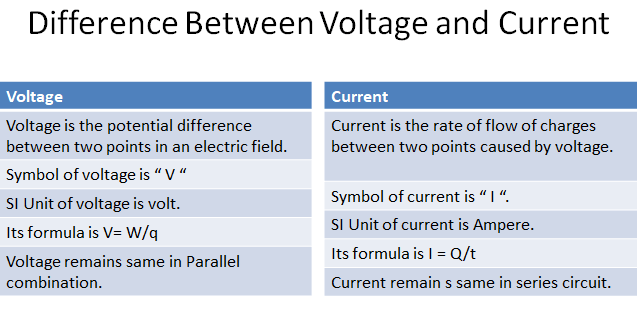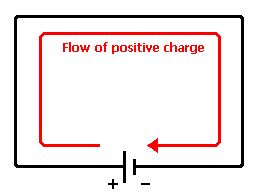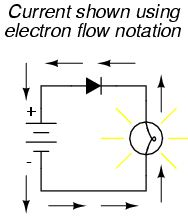# Difference Between Voltage and CurrentThe basic difference between voltage and current is that Voltage is the amount of energy per charge which is required to move electrons from one point to another, while current is the rate of flow of charges.

## What is voltage in electricity?

“Voltage is the amount of energy per charge available to move electrons from one point to another in an electric circuit.”It is the driving force in electric circuits and is what establishes a current. As you have seen, a force of attraction exists between a positive and a negative charge. A certain amount of energy must be exerted, in the form of work to overcome the force and move the charges a given distance apart. All apposite charges possess certain potential energy because of the separation between them. The difference in potential energy per charge is the potential difference or voltage.

Consider a water tank that is supported several feet above the ground. A given amount of energy must be exerted in the form of work to pump water up to fill the tank. Once the water is stored in the tank, it has a certain potential energy which, if released, can be used to perform work. For example, the water can be allowed to fall down a chute to turn a water wheel. The difference in potential energy per charge in electrical terms is called voltage, symbolized by V , and is defined as energy on work W per unit charge(Q).

V=W/Q

Where W is expressed in joules(j) and Q is in coulombs (C).

#### Unit of voltage:

The unit of voltage is volt. It is represented by V.”One volt is the potential difference (voltage) between two points when a joule of energy is used to move one coulomb of charge from point to the other.

#### sources :

A voltage source is a source of electrical potential energy is an electromotive force, more commonly known as voltage.

• ##### Battery:

A battery is a type of voltage source that converts chemical energy into electrical energy. A battery consists of one or more electrochemical cells that are electrically connected. A cell consists of four basic components:

• a positive electrode
• a negative electrode
• electrolyte
• porous separator

the positive electrode as a deficiency of electrons due to chemical reactions, the negative electrode as a surplus of electrons due to chemical reactions, the electrolyte provides a mechanism for charge flow between positive and negative electrodes, and the separator electrically isolates the positive and negative electrodes. A  basic diagram of the battery is shown in fig of battery.

Batteries are divided into two major classes:

1. Primary Batteries
2. secondary Batteries

Primary batteries are used once and discarded because their chemical reactions are irreversible. Secondary batteries can be recharged and reused many times because they are characterized by reversible chemical reactions.

## What is a current?

“Current is the rate of flow of charges through any cross-sectional area.”It is produced due to the flow of either positive charge or negative charge or both of charges at the same time. Its formula is given as: If the charge Q is passing through an area in time t,then-current I will be:

I=Q/t

### Unit of current

SI unit of current is ampere(A).”If a charge of one coulomb passes through a cross-sectional area in one second,then-current is one ampere.”
An electric current is caused by the motion of an electric charge. The motion of electric charge which causes an electric current is due to the flow of charge carriers. In the case of metallic conductors, the charge carriers are electrons. In electrolysis, the current is produced due to the flow of both positive and negative charges.
In the electrolyte, positive ions are attracted to the cathode and negative ions are attracted to the anode. This movement of ions within the electrolyte constitutes an electric current within the internal circuit. fig of electrolysis.

### Current direction

Early scientists regarded an electric current as a flow of positive charge from positive to negative terminal of the battery through an external circuit. Later on, it was found that a current in metallic conductors is actually due to the flow of negative charge carriers called electrons moving in the opposite direction i.e from negative to the positive terminal of the battery, but it is a convention to take the direction of current as the direction in which positive charge flow.
This current is referred to as conventional current. The reason is that it has been found experimentally that a positive charge moving in one direction is equivalent in all external effects to a negative charge moving in the opposite direction. As the current is measured by its external effects so a current due to motion of negative charge, After reversing its direction of flow can be substituted by an equivalent current due to flow of positive charge.
Thus: The conventional current in a circuit is defined as that current which passes from a point at the higher potential to a point at a lower potential as if it represented a movement of positive charges..”While analyzing the electric circuit, we use the direction of the current according to the above-mentioned conventional current. If we wish to refer to the motion of electrons, we use the term electronic current.#### Current through a Metallic Conductor:

In a metal, the valence electrons are not attached to individual atoms but are free to move about within the body. These electrons are known as free electrons. The free electrons are in random motion just like the molecules of a gas in a container and they act as charge carriers in metals.
The speed of randomly moving electrons depends upon temperature. If we consider any section of metallic wire, the rate at which the electrons pass through it from right to left is the same as the rate at which they pass from left to right. fig..as a result, the current through the wire is zero.
If the ends of the wire are connected to a battery, an electric field E will be set up at every point within the wire. The free electrons will now experience a force in the direction opposite to E. As a result of this force, the free electrons acquire motion in the direction of -E. It may be noted that the force experienced by the free electrons does not produce a net acceleration because the electrons keep on colliding with the atoms of the conductor.
The overall effect of these collisions is to transfer the energy of accelerating electrons to the lattice with the result that the electrons acquire a uniform velocity, called the drift velocity in the direction of –E. The drift velocity is of the order 10-3 m/s, whereas the velocity of free electrons at room temperature due to their thermal motion is several hundred kilometers per second.
Thus, when an electric field is established in a conductor, the free electrons modify random motion in such a way that they drift slowly in the direction opposite to the field.
In other words the electrons, in addition to their violent thermal motion, acquire a constant drift velocity due to which a net directed motion of charges take place along the wire and a current begins to flow through it. A steady current is established in a wire when a constant potential difference is maintained across it which generates the requisite electric field E along the wire.

Related topics: